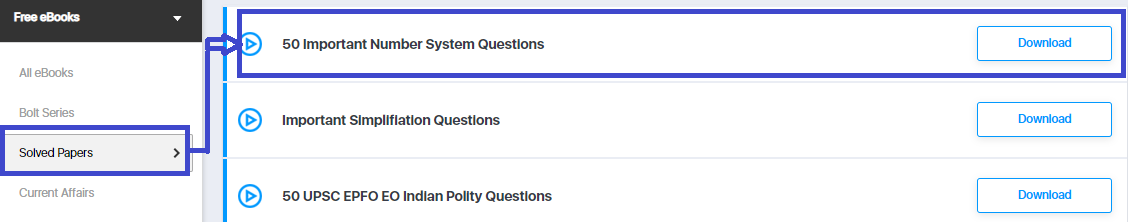# 50 Important Number System Questions [With Solutions] PDF | Download Here

The number system is an essential part of competitive exams like Banking, SSC, Railways & Insurance exams. Wondering what type of Number System questions are asked in different government exams? To help you with your preparation we have brought you the Important Number System Questions in this blog for the practice. We have covered every type of Number System questions that can be asked in upcoming government exams.

If you are preparing for the upcoming government exam. Then you can test your preparation by attempting a free mock test here.Step 1: Click on the above-given download link. You will be taken to Oliveboard’s FREE Ebooks Page.Step 2: Register/Login on to the Free E-Books Page of Oliveboard (It is 100% free, You just enter your valid email id and a password to be able to download the 50 Important Number System Questions).

Step 3: After Logging in, you will see the list of Free Ebooks.

Step 4: Click on the Solved Papers section under All ebooks sections.Step 5: You will be able to download the 50 Important Number System Questions PDF.Let’s have a look at the sample for the Important Number System Questions

Q1) The digits of a two-digit number are in the ratio of 2 : 3 and the number obtained by interchanging the digits is bigger than the original number by 27. What is the original number?

63
2. 48
3. 96
4. 69

Q2) Three consecutive numbers such that twice the first, 3 times the second, and 4 times the third together make 182. The numbers in question are

18, 22 and 23
2. 18, 19 and 20
3. 19, 20 and 21
4. 20, 21 and 22

Want to Solve More Number System Questions? Attempt A Free Mock Test Here.

Q3) A two-digit number is such that the sum of the digits is 11. When the number with the same digits is reversed is subtracted from this number, the difference is 9.What is the number?

1. 23
2. 24
3. 65
4. 14

Q4) One-fifth of a number is equal to 5/8th of another number. If 35 is added to the first number, it becomes four times the second number. Find the second number.

39
2. 70
3. 40
4. 25

Q5) What least number would be subtracted from 427398 so that the remaining number is divisible by 15?

3
2. 10
3. 16
4. 11

Q6) A number consists of two digits. If the digits interchange places and the new number is added to the original number, then the resulting number will be divisible by:

1. 3
2. 5
3. 9
4. 11

## 2. Free Lockdown Live Tests For Upcoming Banking Exams

Here is what you will get with Live Tests.

1. Compete with Friends – Invite Friends to take Daily Live Tests with you.

2. Get All India Standing.

4. Improve your score by +10.

5. Understand All Clerk and PO Level Exams.

Want to increase your score by +10? Attempt daily free lockdown live tests HERE